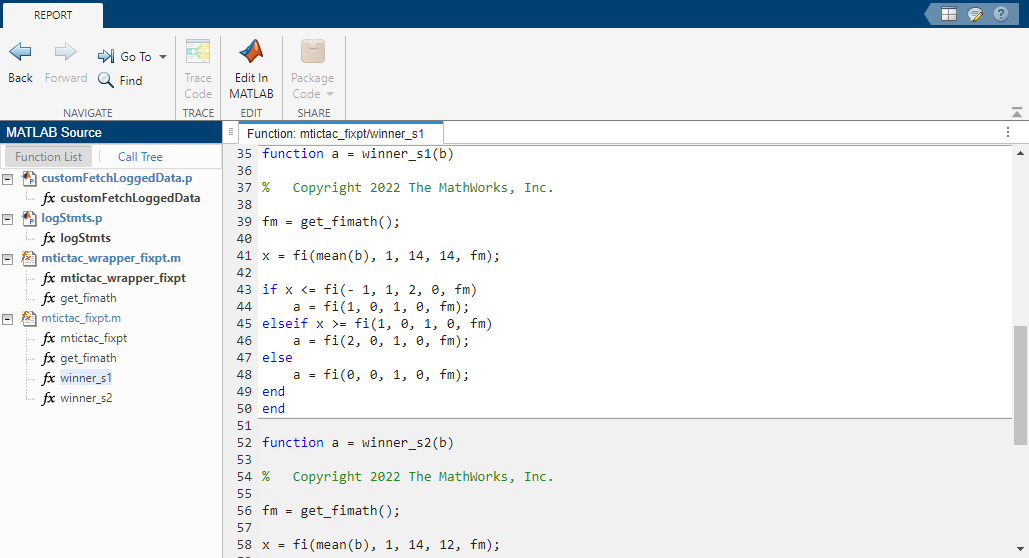## Convert Identical Functions Called with Different Data Types

This example shows how to convert a MATLAB® algorithm containing specialized functions to fixed point using the Fixed-Point Converter app. The example also shows how each instance of the specialized function can have a different data type after conversion.

1. In a local writable folder, create the function `mtictac.m`. The input is a Tic-Tac-Toe game board. The output is the winning player. The output is `0` if there is no winner.

```function a = mtictac(b) % Input is a Tic-Tac-Toe board. % The output is the winning player % or zero if none. % Copyright 2022 The MathWorks, Inc. for i = 1:3 a = winner(b(i,:)); if a > 0 return; end a = winner(b(:,i)); if a > 0 return; end end a = winner(diag(b)); if a>0 return; end a = winner(diag(flip(b)));```

2. In the same folder, create the function, `winner.m`. The `winner.m` function is called by the function `mtictac.m`.

```function a = winner(b) % Copyright 2022 The MathWorks, Inc. x = mean(b); if x <= - 1 a = 1; elseif x >= 1 a = 2; else a = 0; end```

3. In the same folder, create a test file, `mtictac_tb.m`, that calls the `mtictac` function.

```mtictac([-1 0 0; 0 -1 0; 0 0 0]); mtictac([1 0 0; 0 1 0; 0 0 1]); mtictac([0 0 -1; 0 0 -1; 0 0 -1]); ```

4. From the apps gallery, open the Fixed-Point Converter app.

5. Select `mtictac.m` as your entry-point function.

6. Click . On the Define Input Types page, enter the test file that exercises the `mtictac.m` function. Browse to select the `mtictac_tb.m` file. Click .

7. Click . The app generates an instrumented MEX function for your entry-point MATLAB function.

8. Click . Observe that two instances have different data types. The data types for each individual call of the `winner` function can be customized to use different data types.9. Click Convert.

The Fixed-Point Converter app converts the function containing the structures to fixed point and generates the `mtictac_fixpt.m` file.